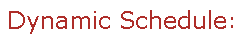Visit Minerva Class Schedule for course dates & times.

# MATH 228 Classical Geometry (3 credits)

Offered by: Mathematics and Statistics (Faculty of Science)

### Overview

Mathematics & Statistics (Sci) : This course is designed to reintroduce classical Euclidean geometry to tomorrow’s teachers. Topics include: Axioms and Euclid’s Elements, the triangle theorem, the Pythagorean Theorem and its extensions, basic constructions and similar triangles, Thales’ theorems and cyclic quadrilaterals, the centers of triangles, the nine-point circle, conic sections and analytic geometry, the prismatoid formula, the Platonic solids, non-Euclidean geometries.

Terms: Fall 2022

Instructors: Cavallo, Alberto (Fall)

• Restrictions: Cannot be taken for credit by students in Mathematics and Statistics programs.

• Not open to students who have taken or are taking MATH 348 or MATH 398.